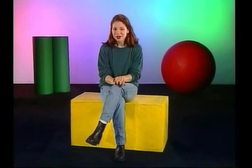PLAY PREVIEW

### Segments in this Video

#### Accelerated Circular Motion(04:26)

FREE PREVIEW

Review uniform circular motion and learn about tangential acceleration. View the total acceleration equation. A sample problem determines tangential acceleration.

#### Accelerated Circular Motion Review(00:52)

Accelerated motion in a circle occurs when an object's velocity changes its magnitude. Hear tangential acceleration properties.

#### Rotational Motion(07:15)

Rotating objects are described by theta, the angle through which they rotate. Learn how to find a radian measurement of a circle, convert degrees to radians, determine angular velocity, and find the linear speed of a point on a globe.

#### Rotational Motion Review(00:50)

An object rotates about an axis at the theta angle, measured in radians. Review equations for angular velocity and linear speed.

#### Linear Momentum (01:57)

Learn about the vector quantity of linear momentum. View a sample problem determining the momentum of a basketball player.

#### Principle of Conservation of Linear Momentum(07:13)

When two particles collide, the final momentum of the system equals the initial momentum of the system. Determine a basketball player's velocity after colliding with another player.

#### Linear Momentum Review(00:28)

Newton derived the Laws of Motion from the Principle of Conservation of Linear Momentum, where the sum of total momenta equals zero and the total momentum after a collision equals the total momentum before it.

#### Credits: Movimiento circular y momentum(01:41)

Credits: Movimiento circular y momentum

For additional digital leasing and purchase options contact a media consultant at 800-257-5126
(press option 3) or sales@films.com.

# Movimiento circular y momentum

Part of the Series : Standard Deviants Español Fisíca Series
 3-Year Streaming Price: \$129.95

Share

### Description

Things really get spinning with a discussion of accelerated circular motion. Then the Standard Deviants cover rotational motion, which is the rotation of objects like planets. Along the way you'll learn about radical radians and other science lingo. Finally, we'll look at linear momentum and its companion, the Principle of Conservation of Linear Momentum. The study of motion in a circle applies to a wide range of activities: from Ferris wheels to Ferraris and technologies from space exploration to machine design. Rotational motion is simple and fun to study, as it involves rotating objects such as tops and orbiting objects such as satellites. Linear and angular momentum problems are explored. Students calculate answers in rads (radians), degrees, and trig functions and use the metric system almost exclusively.

Length: 28 minutes

Item#: BVL155188Closed Captioned